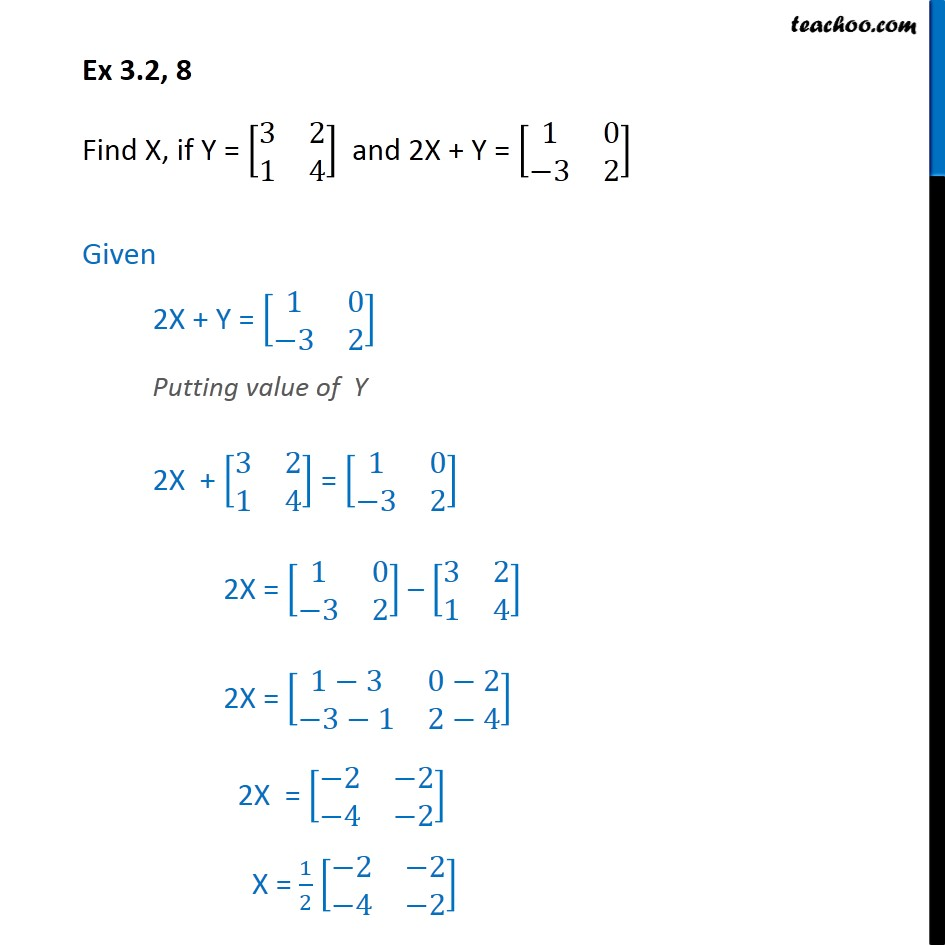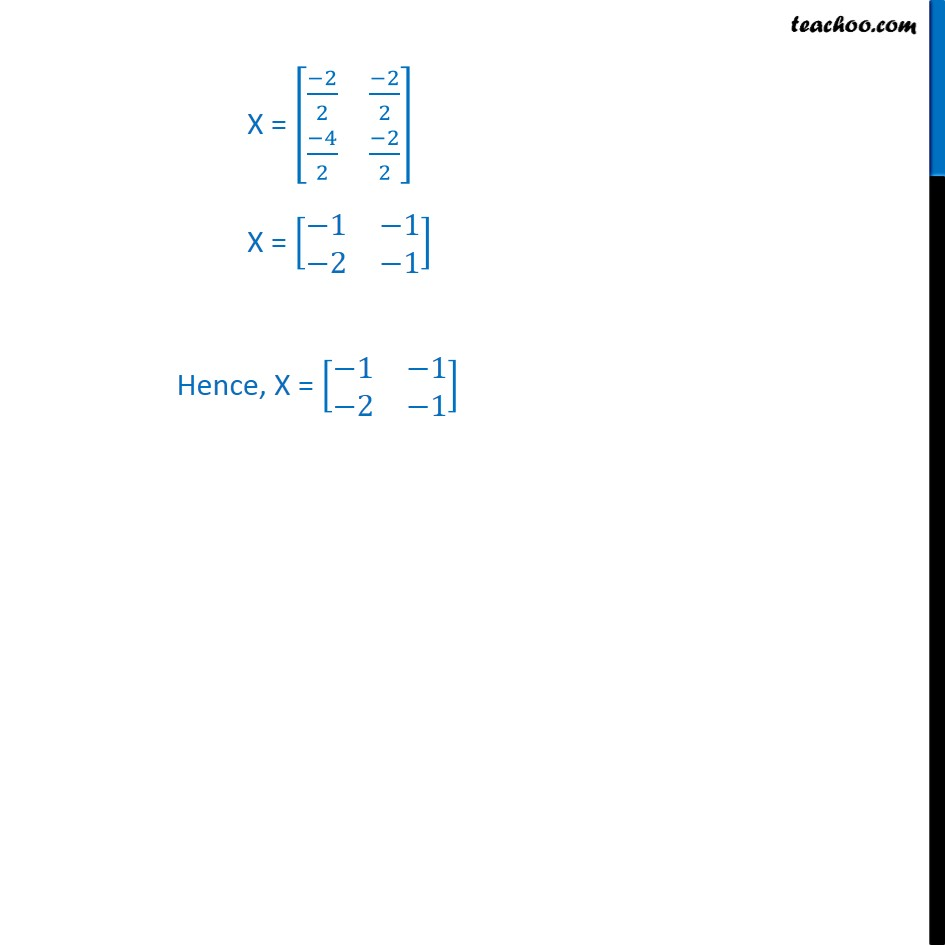Ex 3.2

Chapter 3 Class 12 Matrices
Serial order wiseGet live Maths 1-on-1 Classs - Class 6 to 12

### Transcript

Ex 3.2, 8 Find X, if Y = [■8(3&[email protected]&4)] and 2X + Y = [■8(1&[email protected]−3&2)] Given 2X + Y = [■8(1&[email protected]−3&2)] Putting value of Y 2X + [■8(3&[email protected]&4)] = [■8(1&[email protected]−3&2)] 2X = [■8(1&[email protected]−3&2)] – [■8(3&[email protected]&4)] 2X = [■8(1−3&0−[email protected]−3−1&2−4)] 2X = [■8(−2&−[email protected]−4&−2)] X = 1/2 [■8(−2&−[email protected]−4&−2)] X = [■8((−2)/2&(−2)/[email protected](−4)/2&(−2)/2)] X = [■8(−1&−[email protected]−2&−1)] Hence, X = [■8(−1&−[email protected]−2&−1)]